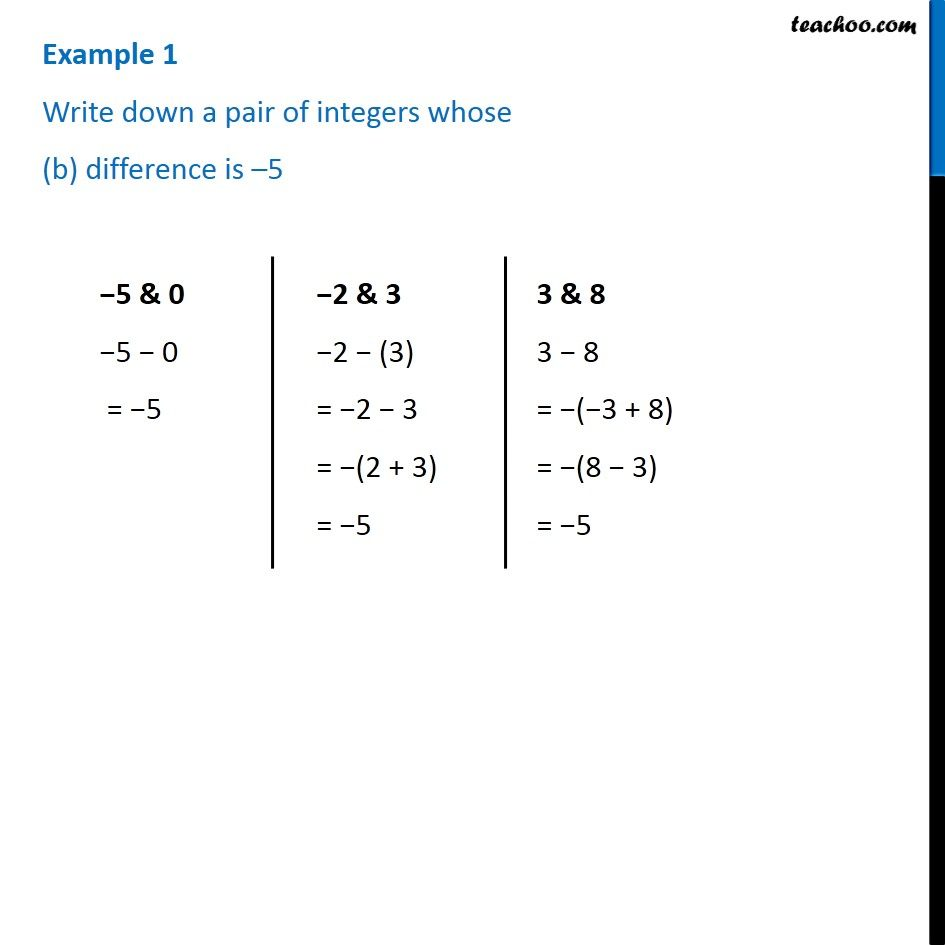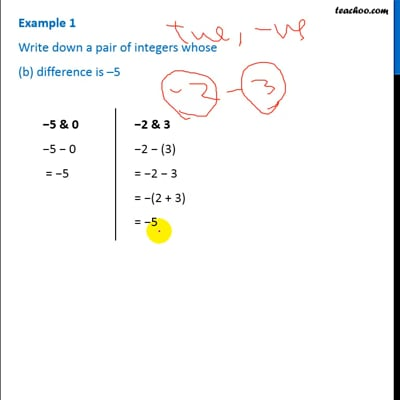Examples

Chapter 1 Class 7 Integers
Serial order wiseThis video is only available for Teachoo black users

Introducing your new favourite teacher - Teachoo Black, at only ₹83 per month

### Transcript

Example 1 Write down a pair of integers whose (b) difference is –5 −5 & 0 −5 − 0 = −5 −2 & 3 −2 − (3) = −2 − 3 = −(2 + 3) = −5 3 & 8 3 − 8 = −(−3 + 8) = −(8 − 3) = −5